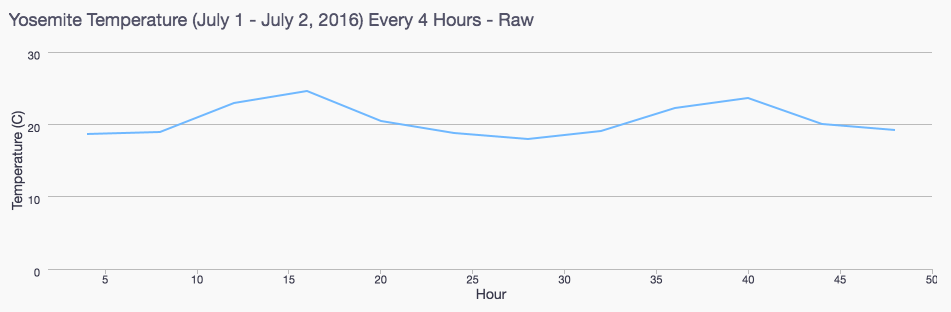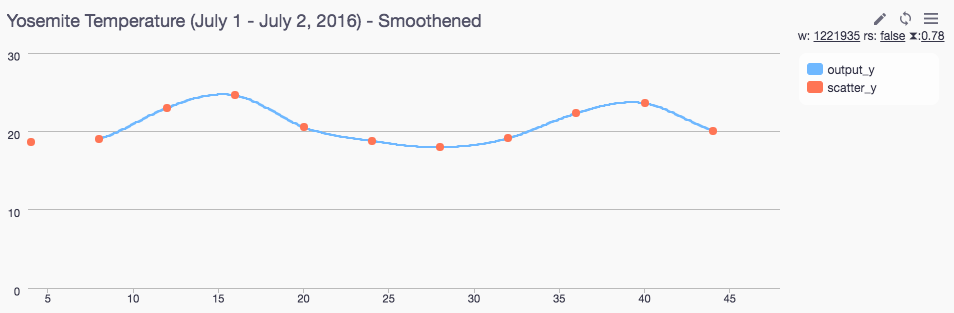No items found.

# Using Spline Interpolation in SQL to Analyze Sparse Data

#### Spline Interpolation using SQL

Splines, piecewise polynomials segmented by discrete points, are known to be a good approximation for many real-world scenarios. Data scientists often use spline interpolation to produce smooth graphs and estimate missing values by “filling in” the space between discrete points of data.

Consider the following graph, which shows the temperature every 4 hours in Yosemite Park in early July.Fig. 1 Sampled Temperature Data in Yosemite

The graph appears jagged as we have data at 4 hour intervals. Spline interpolation can be used to fill in the areas between points while producing a much smoother graph:Fig. 2 Interpolated Temperature Data in Yosemite

Here we explore how to interpolate the data with spline interpolation using just SQL. We make use of Common-Table Expressions (CTEs) and time windowing functions.

#### The Method

Spline interpolation produces a piecewise cubic polynomial function for each pair of points. These distinct polynomial functions join smoothly at each data point. This is distinct from a rolling average, where the line rarely intersects a data point. In practice, a rolling average is good to smooth high variance data, while spline interpolation is used to fill in sparse data. This wikipedia article on spline interpolation is a good introduction to the topic, and we’ll be referring to the various equations in that article here.

Our primary task is to solve for the variables in 3 equations - equation 15 constrains the polynomial functions to be smooth at each data point, and equations 16 and 17 constrain the curvature at the end points to be straight lines.

As the article shows, these 3 equations can be represented in matrix form. Conveniently, the coefficient matrix is a tridiagonal matrix which can be solved using the Thomas Algorithm, as explained here.

The interpolation algorithm normally uses the entire data set to determine the polynomial functions. In our implementation, however, we consider a subset of 4 nearby data points when determining the coefficients of each polynomial (also called windowing).

The resulting matrices for our study (n=4) are useful for explanatory purposes and produce the smooth graphs we are looking for, but you may find the need to increase the window size when using real world data.

#### The Source Data

To begin, we first download temperature data from the National Oceanic and Atmospheric Administration (NOAA) website, cut it down to the first 2 days of July 2017, and insert into a table as follows:

create table temperatures (hour integer, temperature double precision);
insert into temperatures (hour, temperature) values
(1,19.5),
(2,19.5),
(3,19.7),

(176,14.6);

In order to simulate a rough sampling, we extract the values every 4 hours:

with xy as (
select
cast(hour as double precision) as x
, temperature as y
from temperatures
where
cast(hour as integer) % 4 = 0
order by
hour
limit
12
)

This is what we plot to get the graph in fig. 1 above.

#### Generating the Series

In order to interpolate we need additional points in the x-axis to produce a higher resolution. We join with our original data set so we can see the actual data set. We use a recursive function here to add ad a point for each 1/10 of an hour.

with recursive
, x_series(input_x) as (
select
min(x)
from
xy
union all
select
input_x + 0.1
from
x_series
where
input_x + 0.1 < (select max(x) from x)
)
, x_coordinate as (
select
input_x
, max(x) over(order by input_x) as previous_x
from
x_series
left join
xy on abs(x_series.input_x - xy.x) < 0.001
)

#### Preparing the Input Variables - The 4 Points in Each Window

As mentioned, we will use a window of 4 data points around each data point. This is easy to do by creating columns for the (x, y) coordinate for each of the 4 data points by leveraging the lag and lead functions.

fullxy as (
select
x
, y
, lag(x, 1) over (order by x) as x0
, lag(y, 1) over (order by x) as y0
, x as x1
, y as y1
, lead(x, 1) over (order by x) as x2
, lead(y, 1) over (order by x) as y2
, lead(x, 2) over (order by x) as x3
, lead(y, 2) over (order by x) as y3
from
xy
order by
x
)

This produces a table like the following:

As we can see, for each point (x, y), we also duplicate the (x, y) coordinates of the point before it (x0, y0) and the two points following it (x2, y2) and (x3, y3), for a total of 4 data points per row.

#### Producing the Tridiagonal Coefficients

, tridiagonal as (
select
*
, 3*(y1-y0) /power(x1-x0, 2) as d0
, 3*((y1-y0)/(power(x1-x0, 2)) + (y2-y1)/(power(x2-x1, 2))) as d1
, 3*((y2-y1)/(power(x2-x1, 2)) + (y3-y2)/(power(x3-x2, 2))) as d2
, 3*(y3-y2) /power(x3-x2, 2) as d3
, 1 / (x1-x0) as a1
, 1 / (x2-x1) as a2
, 1 / (x3-x2) as a3
, 2 / (x1-x0) as b0
, 2 * (1/(x1-x0) + 1/(x2-x1)) as b1
, 2 * (1/(x2-x1) + 1/(x3-x2)) as b2
, 2 / (x3-x2) as b3
, 1 / (x1-x0) as c0
, 1 / (x2-x1) as c1
, 1 / (x3-x2) as c2
from fullxy
order by x)

Now, for each row, we have the necessary a, b, c and d vectors for the tridiagonal matrix.

#### Finding the Coefficients for the Tridiagonal Matrix Using the Thomas Algorithm

The Thomas Algorithm can now be used to solve the tridiagonal matrix we created. The algorithm uses a series of 'sweeps' across the matrix to find a solution. First there is a forward sweep, where the c prime and d prime values are calculated, and then a backwards substitution, where the solution slope vector is solved. We unroll the calculation into distinct sweeps and solve in a series of CTEs behaving like nested subqueries.

forward_sweep_1 as (
select
*
, c0 / b0 as c_prime_0
, c1 / (b1 - a1 * c0 / b0) as c_prime_1
, c2 / (b2 - a2 * (c1 / (b1 - a1 * c0 / b0))) as c_prime_2
, d0 / b0 as d_prime_0
, (d1 - a1 * d0 / b0) / (b1 - a1 * c0 / b0) as d_prime_1
from
tridiagonal
)
, forward_sweep_2 as (
select
*
, (d2 - a2 * d_prime_1) / (b2 - a2 * c_prime_1) as d_prime_2
, (d3 - a3 * ((d2 - a2 * d_prime_1) / (b2 - a2 * c_prime_1)))
/ (b3 - a3 * c_prime_2) as d_prime_3
from
forward_sweep_1
)
, backwards_substitution_1 as (
select
*
, d_prime_3 as k3
, d_prime_2 - c_prime_2 *(d_prime_3) as k2
, d_prime_1 - c_prime_1 * (d_prime_2 - c_prime_2 *(d_prime_3)) as k1
from
forward_sweep_2
)
, backwards_substitution_2 as (
select
*
, d_prime_0 - c_prime_0 * k1 as k0
from
backwards_substitution_1
)

#### Determining the Polynomial Coefficients

Now, we’re ready to determine the polynomial coefficients a and b (equations 10 & 11)

, coefficients as (
select
x, y, x0, y0, x1, y1, x2, y2, k0, k1, k2, k3
k1  * (x2-x1) - (y2-y1)  as a,
-k2 * (x2-x1) + (y2-y1) as b
from
backwards_substitution_2
)

#### Final Step: Calculating the Temperature at Each Interpolated Point

Here we calculate t (equation 2) and output y values (equation 1).

, interpolated_table as (
select
*
, (input_x - x1) / (x2 - x1) as t
from
x_coordinate,
coefficients
where
abs(coefficients.x1 - x_coordinate.previous_x) < 0.001
)
, final_table as (
select
*
, (1-t) * y1 + t*y2 + t*(1-t)*(a*(1-t)+b*t) as output_y
from
interpolated_table
order by input_x
)

The following is the final select clause. output_y contains the interpolated values. We also include the original (x, y) coordinate as well as scatter_y so we can display the original data points on a scatter plot.

select
round(cast(input_x as numeric), 2) as input_x
, x
, y
, round(cast(output_y as numeric), 2) as output_y
, case
when abs(input_x - x) < 0.01 then y
else null
end as scatter_y
from
final_table
order by
x

The output is shown below:

#### Final Considerations

And there you have it! Using only SQL, we have used spline interpolation to generate smooth graphs. For the sake of brevity, we excluded data points on the left and right most of the data set but they can be added back into your analysis. Using splines for your graphs makes time series easier to read and pushes the limits of SQL.

Happy scripting!

#### Want to discuss this article? Join the Periscope Data Community!